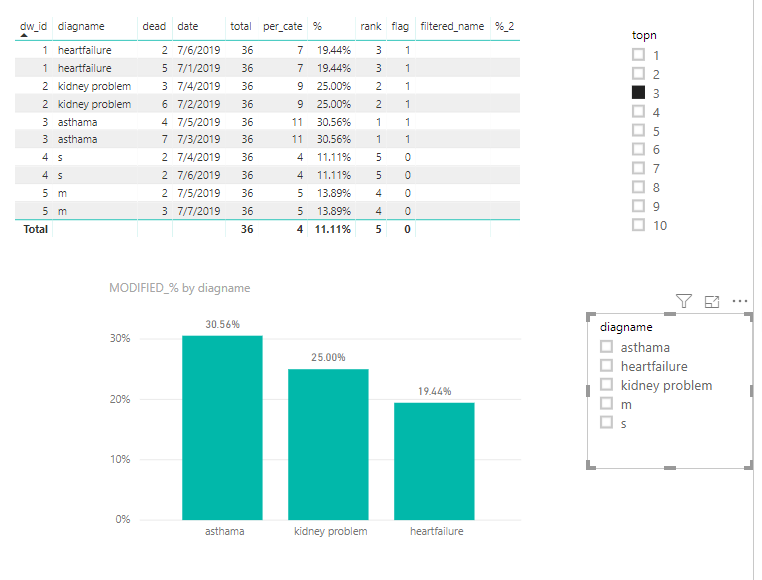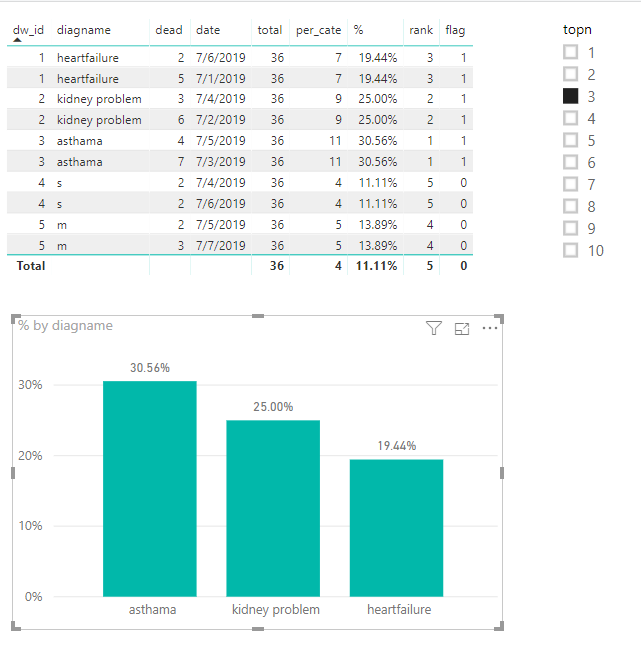cancel
Showing results for
Did you mean:Anonymous
Not applicable

## ignore the top N filter for calculation of percentage value

i have the simple scnerio as below .

1           heartfailure                                 2                     06/07/2019

2              kidney problem                     3                     04/07/2019

3             asthama                                       4                     05/07/19

i have like 100 category of dignosisname and i have used the bar chart to show only 10 deads during that period . where i have the date as slicer and dw_id in the filterpane . i need to have the measure of dead % when i categorize by diganosisname  = dead of that category/total dead . But when i strict the top 10 it is stricting the total count for that tile and % is showing the wrong value . For example i have total dead for the time period of jan 2019 is 400 and top 10 dead is limiting to 300 count . Value is being calculated on 300 instead of 400 . Is there any way i can ignore the top N filter for calculation ?

currently i am using measure like below :

8 REPLIES 8Community Support

Hi @Anonymous

Modified measure "total" as below

`total = CALCULATE(SUM(Sheet6[dead]),ALLSELECTED(Sheet6))`Best Regards
Maggie

Community Support Team _ Maggie Li
If this post helps, then please consider Accept it as the solution to help the other members find it more quickly.Anonymous
Not applicable

@v-juanli-msft : To explain further . Here are the two scenerios

1) one with filter : In below way 100% is correct .

2) without filter : But this case is failing like  below in the inital reply suggested . I am seeing asthama 40.74 % which is wrong . can i get 11/36 =30.5 % . I know that you suggested to use ALL instead of ALLselected but in this case case 1) is failing . I need both the cases to work . Can i have the formula written in such a way ?Community Support

Hi @Anonymous

Based on the measures above

```total = CALCULATE(SUM(Sheet6[dead]),ALL(Sheet6))

% = [per_cate]/[total]

rank = RANKX(ALLSELECTED(Sheet6),Sheet6[%],,DESC,Dense)

flag = IF([rank]<=SELECTEDVALUE('topn table'[topn]),1,0)```

Plus the steps below:

1.Create a table

`diagname table = VALUES(Sheet6[diagname])`

Leave it alone, don't connect to other tables

2. create measures in Sheet4 (my table)

```total_2 = CALCULATE(SUM(Sheet6[dead]),ALLSELECTED(Sheet6))

filtered_name = SELECTEDVALUE('diagname table'[diagname])

%_2 = IF([filtered_name]=MAX(Sheet6[diagname]),[per_cate]/[total_2])

MODIFIED_% = IF(ISFILTERED('diagname table'[diagname]),[%_2],[%])```Best Regards
Maggie

Community Support Team _ Maggie Li
If this post helps, then please consider Accept it as the solution to help the other members find it more quickly.Anonymous
Not applicable

@v-juanli-msft : If you see below screenshot when diagname filtered by asthama i was able to see the chart  but when  i apply both asthama and  hearfailure i am not seeing any data in the chart . Can you help here please :Community Support

Hi @Anonymous

1.Create a new table not related to any other table( have no relationship between this table and other tables)Add [topn] from this table to a slicer

2. create measures in main table

```total = CALCULATE(SUM(Sheet6[dead]),ALLSELECTED(Sheet6))

% = [per_cate]/[total]

rank = RANKX(ALLSELECTED(Sheet6),Sheet6[%],,DESC,Dense)

flag = IF([rank]<=SELECTEDVALUE('topn table'[topn]),1,0)```

3. add [flag] to the visual level filter of the column chartBest Regards
Maggie

Community Support Team _ Maggie Li
If this post helps, then please consider Accept it as the solution to help the other members find it more quickly.Anonymous
Not applicable

hi

Thanks for responding you almost reached my requirement but is not  exactly what i am looking for .

to explain that in the screenshot below i have total dead is 36 and as per below i selected top 3 per say . My user is saying he need the formula in below way % for asthama should be = 11/36 =30.5 % but what you are showing is 40.74 .

Reason is total dead is being restricted to top 3 even if we have 5 ranks . But i need the percentage to be calculated in above way . Is that possible if i apply flag as you shown ?Community Support

Hi @Anonymous

Modify formula

`total = CALCULATE(SUM(Sheet6[dead]),ALL(Sheet6))`Best Regards
Maggie

Community Support Team _ Maggie Li
If this post helps, then please consider Accept it as the solution to help the other members find it more quickly.Anonymous
Not applicable

@v-juanli-msft : Thanks for response but still my user request is not solved . Below is the screeshot which i am attaching with clear requirement . if i applied the filter % should be 100 % and without filter it is giving correct value .Below description explains the clearly of requirement: